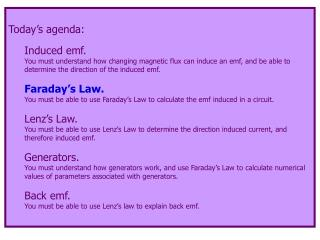# Today’s agenda: Induced emf. - PowerPoint PPT PresentationDownload PresentationToday’s agenda: Induced emf.

Today’s agenda: Induced emf.Download Presentation## Today’s agenda: Induced emf.

- - - - - - - - - - - - - - - - - - - - - - - - - - - E N D - - - - - - - - - - - - - - - - - - - - - - - - - - -
##### Presentation Transcript

1. Today’s agenda: Induced emf. You must understand how changing magnetic flux can induce an emf, and be able to determine the direction of the induced emf. Faraday’s Law. You must be able to use Faraday’s Law to calculate the emf induced in a circuit. Lenz’s Law. You must be able to use Lenz’s Law to determine the direction induced current, and therefore induced emf. Generators. You must understand how generators work, and use Faraday’s Law to calculate numerical values of parameters associated with generators. Back emf. You must be able to use Lenz’s law to explain back emf.

2. We can quantify the induced emf described qualitatively in the previous section of this lecture by using magnetic flux. Experimentally, if the flux through N loops of wire changes by dB in a time dt, the induced emf is *Faraday’s Law of Magnetic Induction Faraday’s law of induction is one of the fundamental laws of electricity and magnetism. I wonder why the – sign… Your text, page 301, shows how to determine the direction of the induced emf. Argh! Lenz’s Law, coming soon, is much easier. *Well, one expression of Faraday’s Law

3. Faraday’s Law of Magnetic Induction In the equation is the magnetic flux. This is another expression of Faraday’s Law: We’ll use this version in the next lecture.

4. N S I v + - Example: move a magnet towards a coil of wire. N=5 turns A=0.002 m2

5. Possible homework hint: if B varies but loop  B. Ways to induce an emf:  change B  change the area of the loop in the field Possible homework hint: for a circular loop, C=2R, so A=r2=(C/2)2=C2/4, so you can express d(BA)/dt in terms of dC/dt.

6. Ways to induce an emf (continued):  changing current changes B through conducting loop a I B b Possible Homework Hint. The magnetic field is not uniform through the loop, so you can’t use BA to calculate the flux. Take an infinitesimally thin strip. Then the flux is d = BdAstrip. Integrate from a to b to get the flux through the strip.

7. =90 =45 =0 Ways to induce an emf (continued):  change the orientation of the loop in the field